This is “Essential Skills 1”, section 1.9 from the book Principles of General Chemistry (v. 1.0M). For details on it (including licensing), click here.

For more information on the source of this book, or why it is available for free, please see the project's home page. You can browse or download additional books there. To download a .zip file containing this book to use offline, simply click here.

Has this book helped you? Consider passing it on:
Creative Commons supports free culture from music to education. Their licenses helped make this book available to you.
DonorsChoose.org helps people like you help teachers fund their classroom projects, from art supplies to books to calculators.

## 1.9 Essential Skills 1

### Topics

• Measurement
• Scientific Notation
• Significant Figures
• Accuracy and Precision

This section describes some of the fundamental mathematical skills you will need to complete the questions and problems in this text. For some of you, this discussion will serve as a review, whereas others may be encountering at least some of the ideas and techniques for the first time. We will introduce other mathematical skills in subsequent Essential Skills sections as the need arises. Be sure you are familiar with the topics discussed here before you start the Chapter 1 "Introduction to Chemistry" problems.

## Instruments of Measurement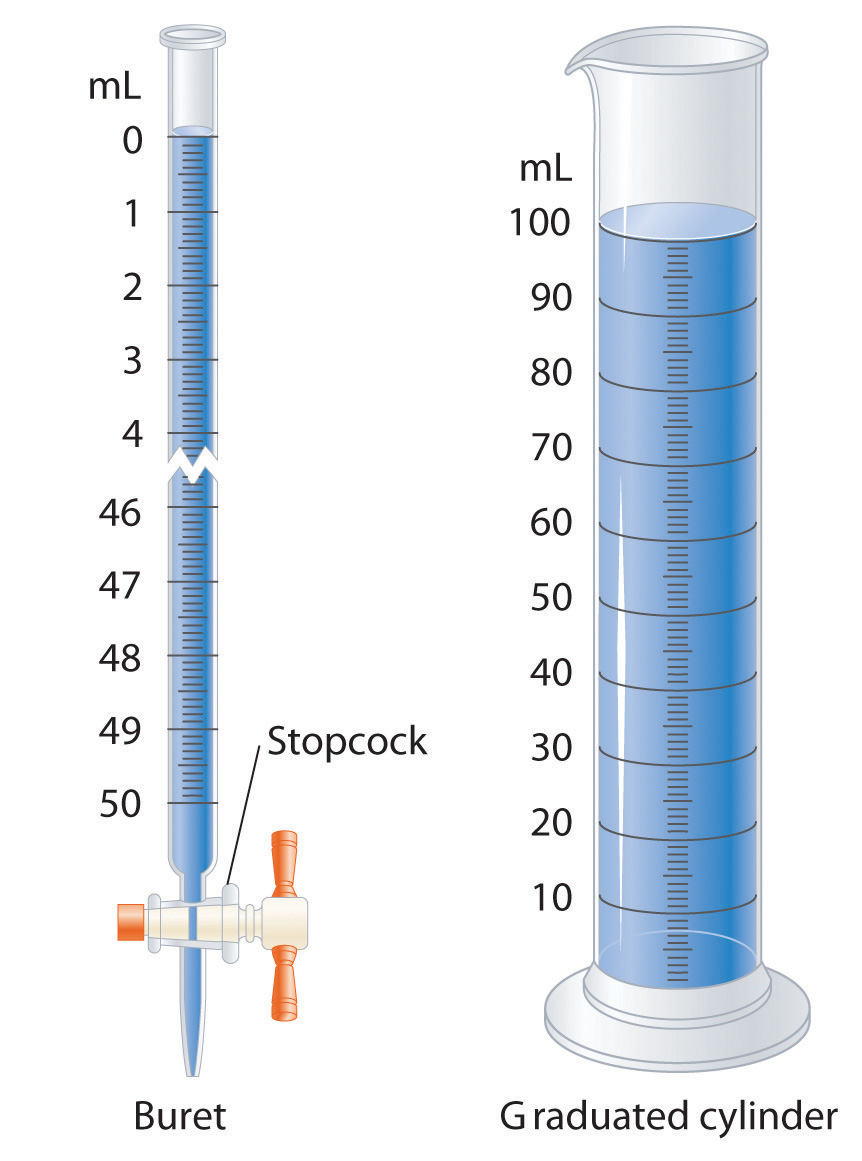Graduated glassware is used to deliver variable volumes of liquid.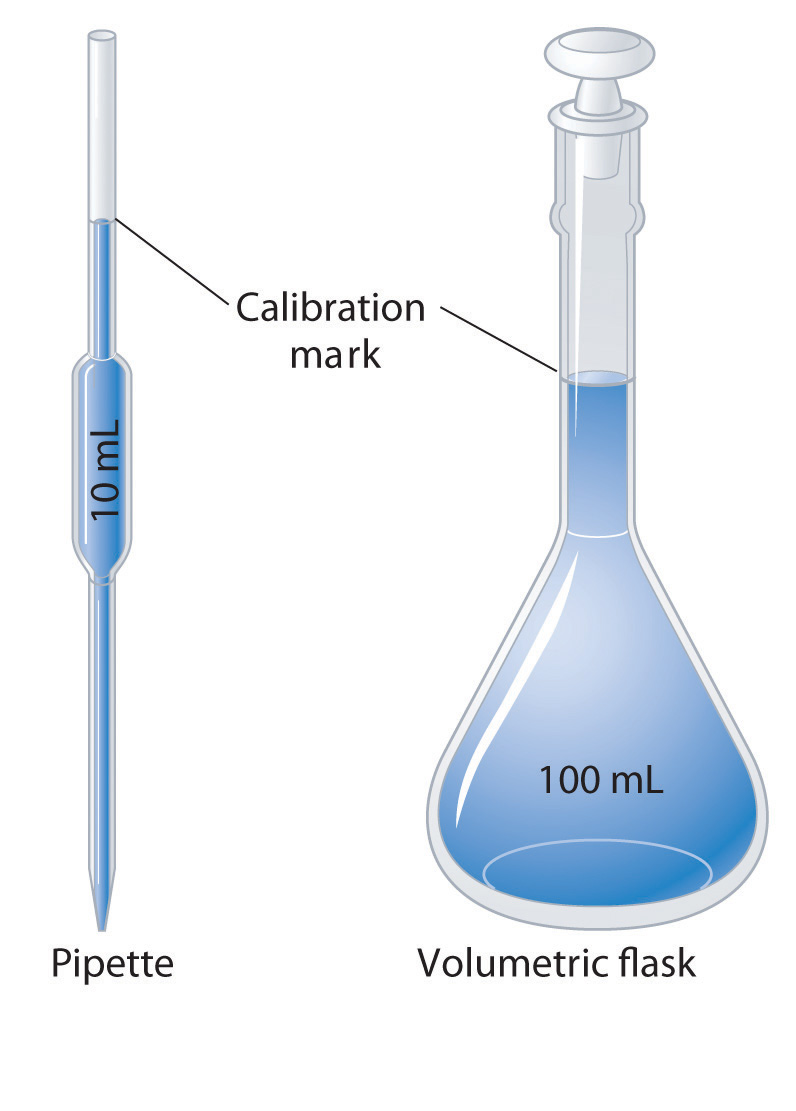Volumetric glassware is used to deliver (pipette) or contain (volumetric flask) a single volume accurately when filled to the calibration mark.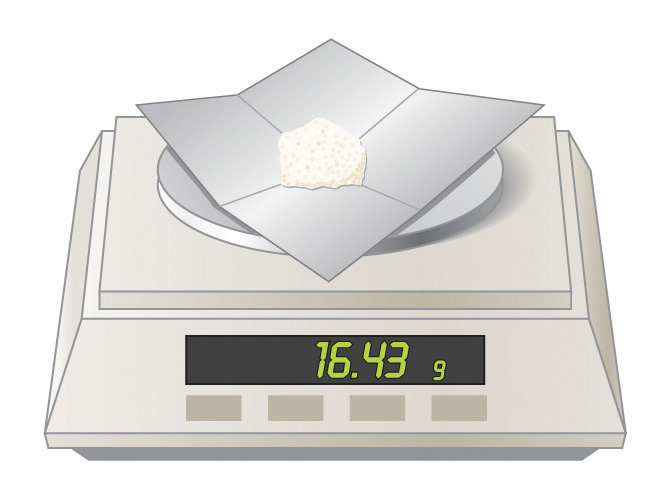A balance is used to measure mass.

A variety of instruments are available for making direct measurements of the macroscopic properties of a chemical substance. For example, we usually measure the volumeThe amount of space occupied by a sample of matter. of a liquid sample with pipettes, burets, graduated cylinders, and volumetric flasks, whereas we usually measure the mass of a solid or liquid substance with a balance. Measurements on an atomic or molecular scale, in contrast, require specialized instrumentation, such as the mass spectrometer described in Section 1.6 "Isotopes and Atomic Masses".

## SI Units

All reported measurements must include an appropriate unit of measurement because to say that a substance has “a mass of 10,” for example, does not tell whether the mass was measured in grams, pounds, tons, or some other unit. To establish worldwide standards for the consistent measurement of important physical and chemical properties, an international body called the General Conference on Weights and Measures devised the Système internationale d’unités (or SI)A system of units based on metric units that requires measurements to be expressed in decimal form. There are seven base units in the SI system.. The International System of Units is based on metric units and requires that measurements be expressed in decimal form. Table 1.7 "SI Base Units" lists the seven base units of the SI system; all other SI units of measurement are derived from them.

By attaching prefixes to the base unit, the magnitude of the unit is indicated; each prefix indicates that the base unit is multiplied by a specified power of 10. The prefixes, their symbols, and their numerical significance are given in Table 1.8 "Prefixes Used with SI Units". To study chemistry, you need to know the information presented in Table 1.7 "SI Base Units" and Table 1.8 "Prefixes Used with SI Units".

Table 1.7 SI Base Units

Base Quantity Unit Name Abbreviation
mass kilogram kg
length meter m
time second s
temperature kelvin K
electric current ampere A
amount of substance mole mol
luminous intensity candela cd

Table 1.8 Prefixes Used with SI Units

Prefix Symbol Value Power of 10 Meaning
tera T 1,000,000,000,000 1012 trillion
giga G 1,000,000,000 109 billion
mega M 1,000,000 106 million
kilo k 1000 103 thousand
hecto h 100 102 hundred
deca da 10 101 ten
1 100 one
deci d 0.1 10−1 tenth
centi c 0.01 10−2 hundredth
milli m 0.001 10−3 thousandth
micro μ 0.000001 10−6 millionth
nano n 0.000000001 10−9 billionth
pico p 0.000000000001 10−12 trillionth
femto f 0.000000000000001 10−15 quadrillionth

## Units of Mass, Volume, and Length

The units of measurement you will encounter most frequently in chemistry are those for mass, volume, and length. The basic SI unit for mass is the kilogram (kg), but in the laboratory, mass is usually expressed in either grams (g) or milligrams (mg): 1000 g = 1 kg, 1000 mg = 1 g, and 1,000,000 mg = 1 kg. Units for volume are derived from the cube of the SI unit for length, which is the meter (m). Thus the basic SI unit for volume is cubic meters (length × width × height = m3). In chemistry, however, volumes are usually reported in cubic centimeters (cm3) and cubic decimeters (dm3) or milliliters (mL) and liters (L), although the liter is not an SI unit of measurement. The relationships between these units are as follows:

1 L = 1000 mL = 1 dm3 1 mL = 1 cm3 1000 cm3 = 1 L

## Scientific Notation

Chemists often work with numbers that are exceedingly large or small. For example, entering the mass in grams of a hydrogen atom into a calculator requires a display with at least 24 decimal places. A system called scientific notationA system that expresses numbers in the form N × 10n, where N is greater than or equal to 1 and less than 10 (1 ≤ N < 10) and n is an integer that can be either positive or negative (100 = 1). The purpose of scientific notation is to simplify the manipulation of numbers with large or small magnitudes. avoids much of the tedium and awkwardness of manipulating numbers with large or small magnitudes. In scientific notation, these numbers are expressed in the form

N × 10n

where N is greater than or equal to 1 and less than 10 (1 ≤ N < 10), and n is a positive or negative integer (100 = 1). The number 10 is called the base because it is this number that is raised to the power n. Although a base number may have values other than 10, the base number in scientific notation is always 10.

A simple way to convert numbers to scientific notation is to move the decimal point as many places to the left or right as needed to give a number from 1 to 10 (N). The magnitude of n is then determined as follows:

• If the decimal point is moved to the left n places, n is positive.
• If the decimal point is moved to the right n places, n is negative.

Another way to remember this is to recognize that as the number N decreases in magnitude, the exponent increases and vice versa. The application of this rule is illustrated in Skill Builder ES1.

### Skill Builder ES1

Convert each number to scientific notation.

1. 637.8
2. 0.0479
3. 7.86
4. 12,378
5. 0.00032
6. 61.06700
7. 2002.080
8. 0.01020

Solution

1. To convert 637.8 to a number from 1 to 10, we move the decimal point two places to the left:

$6 3 ↖ 7 ↖ .8$

Because the decimal point was moved two places to the left, n = 2. In scientific notation, 637.8 = 6.378 × 102.

2. To convert 0.0479 to a number from 1 to 10, we move the decimal point two places to the right:

$0. 0 ↗ 4 ↗ 79$

Because the decimal point was moved two places to the right, n = −2. In scientific notation, 0.0479 = 4.79 × 10−2.

3. 7.86 × 100: this is usually expressed simply as 7.86. (Recall that 100 = 1.)
4. 1.2378 × 104; because the decimal point was moved four places to the left, n = 4.
5. 3.2 × 10−4; because the decimal point was moved four places to the right, n = −4.
6. 6.106700 × 101: this is usually expressed as 6.1067 × 10.
7. 2.002080 × 103
8. 1.020 × 10−2

## Addition and Subtraction

Before numbers expressed in scientific notation can be added or subtracted, they must be converted to a form in which all the exponents have the same value. The appropriate operation is then carried out on the values of N. Skill Builder ES2 illustrates how to do this.

### Skill Builder ES2

Carry out the appropriate operation on each number and then express the answer in scientific notation.

1. (1.36 × 102) + (4.73 × 103)
2. (6.923 × 10−3) − (8.756 × 10−4)

Solution

1. Both exponents must have the same value, so these numbers are converted to either (1.36 × 102) + (47.3 × 102) or (0.136 × 103) + (4.73 × 103). Choosing either alternative gives the same answer, reported to two decimal places:

(1.36 × 102) + (47.3 × 102) = (1.36 + 47.3) × 102 = 48.66 × 102 = 4.87 × 103 (0.136 × 103) + (4.73 × 103) = (0.136 + 4.73) × 103 = 4.87 × 103

In converting 48.66 × 102 to scientific notation, n has become more positive by 1 because the value of N has decreased.

2. Converting the exponents to the same value gives either (6.923 × 10−3) − (0.8756 × 10−3) or (69.23 × 10−4) − (8.756 × 10−4). Completing the calculations gives the same answer, expressed to three decimal places:

(6.923 × 10−3) − (0.8756 × 10−3) = (6.923 − 0.8756) × 10−3 = 6.047 × 10−3 (69.23 × 10−4) − (8.756 × 10−4) = (69.23 − 8.756) × 10−4 = 60.474 × 10−4 = 6.047 × 10−3

## Multiplication and Division

When multiplying numbers expressed in scientific notation, we multiply the values of N and add together the values of n. Conversely, when dividing, we divide N in the dividend (the number being divided) by N in the divisor (the number by which we are dividing) and then subtract n in the divisor from n in the dividend. In contrast to addition and subtraction, the exponents do not have to be the same in multiplication and division. Examples of problems involving multiplication and division are shown in Skill Builder ES3.

### Skill Builder ES3

Perform the appropriate operation on each expression and express your answer in scientific notation.

1. (6.022 × 1023)(6.42 × 10−2)
2. $1.67 × 10 − 24 9.12 × 10 − 28$
3. $( 6.63 × 10 − 34 ) ( 6.0 × 10 ) 8.52 × 10 − 2$

Solution

1. In multiplication, we add the exponents:

2. In division, we subtract the exponents:

3. This problem has both multiplication and division:

## Significant Figures

No measurement is free from error. Error is introduced by (1) the limitations of instruments and measuring devices (such as the size of the divisions on a graduated cylinder) and (2) the imperfection of human senses. Although errors in calculations can be enormous, they do not contribute to uncertainty in measurements. Chemists describe the estimated degree of error in a measurement as the uncertaintyThe estimated degree of error in a measurement. The degree of uncertainty in a measurement can be indicated by reporting all significant figures plus one. of the measurement, and they are careful to report all measured values using only significant figuresNumbers that describe the value without exaggerating the degree to which it is known to be accurate., numbers that describe the value without exaggerating the degree to which it is known to be accurate. Chemists report as significant all numbers known with absolute certainty, plus one more digit that is understood to contain some uncertainty. The uncertainty in the final digit is usually assumed to be ±1, unless otherwise stated.

The following rules have been developed for counting the number of significant figures in a measurement or calculation:

1. Any nonzero digit is significant.
2. Any zeros between nonzero digits are significant. The number 2005, for example, has four significant figures.
3. Any zeros used as a placeholder preceding the first nonzero digit are not significant. So 0.05 has one significant figure because the zeros are used to indicate the placement of the digit 5. In contrast, 0.050 has two significant figures because the last two digits correspond to the number 50; the last zero is not a placeholder. As an additional example, 5.0 has two significant figures because the zero is used not to place the 5 but to indicate 5.0.
4. When a number does not contain a decimal point, zeros added after a nonzero number may or may not be significant. An example is the number 100, which may be interpreted as having one, two, or three significant figures. (Note: treat all trailing zeros in exercises and problems in this text as significant unless you are specifically told otherwise.)
5. Integers obtained either by counting objects or from definitions are exact numbersAn integer obtained either by counting objects or from definitions (e.g., 1 in. = 2.54 cm). Exact numbers have infinitely many significant figures., which are considered to have infinitely many significant figures. If we have counted four objects, for example, then the number 4 has an infinite number of significant figures (i.e., it represents 4.000…). Similarly, 1 foot (ft) is defined to contain 12 inches (in), so the number 12 in the following equation has infinitely many significant figures:
1 ft = 12 in

An effective method for determining the number of significant figures is to convert the measured or calculated value to scientific notation because any zero used as a placeholder is eliminated in the conversion. When 0.0800 is expressed in scientific notation as 8.00 × 10−2, it is more readily apparent that the number has three significant figures rather than five; in scientific notation, the number preceding the exponential (i.e., N) determines the number of significant figures. Skill Builder ES4 provides practice with these rules.

### Skill Builder ES4

Give the number of significant figures in each. Identify the rule for each.

1. 5.87
2. 0.031
3. 52.90
4. 00.2001
5. 500
6. 6 atoms

Solution

1. three (rule 1)
2. two (rule 3); in scientific notation, this number is represented as 3.1 × 10−2, showing that it has two significant figures.
3. four (rule 3)
4. four (rule 2); this number is 2.001 × 10−1 in scientific notation, showing that it has four significant figures.
5. one, two, or three (rule 4)
6. infinite (rule 5)

### Skill Builder ES5

Which measuring apparatus would you use to deliver 9.7 mL of water as accurately as possible? To how many significant figures can you measure that volume of water with the apparatus you selected?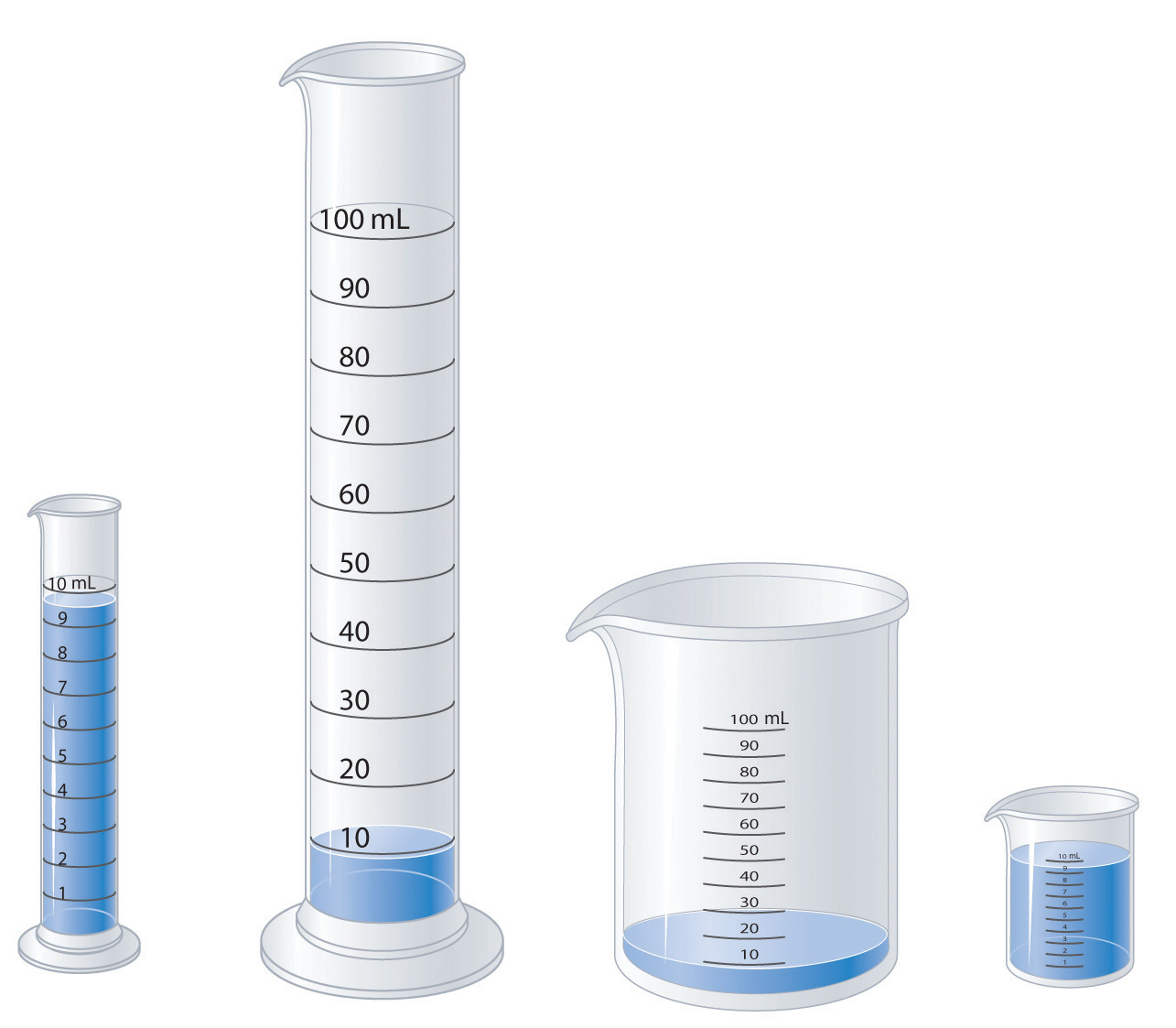Solution

Use the 10 mL graduated cylinder, which will be accurate to two significant figures.

Mathematical operations are carried out using all the digits given and then rounding the final result to the correct number of significant figures to obtain a reasonable answer. This method avoids compounding inaccuracies by successively rounding intermediate calculations. After you complete a calculation, you may have to round the last significant figure up or down depending on the value of the digit that follows it. If the digit is 5 or greater, then the number is rounded up. For example, when rounded to three significant figures, 5.215 is 5.22, whereas 5.213 is 5.21. Similarly, to three significant figures, 5.005 kg becomes 5.01 kg, whereas 5.004 kg becomes 5.00 kg. The procedures for dealing with significant figures are different for addition and subtraction versus multiplication and division.

When we add or subtract measured values, the value with the fewest significant figures to the right of the decimal point determines the number of significant figures to the right of the decimal point in the answer. Drawing a vertical line to the right of the column corresponding to the smallest number of significant figures is a simple method of determining the proper number of significant figures for the answer:

$3240.7 + 21.2 36 3261.9 36$

The line indicates that the digits 3 and 6 are not significant in the answer. These digits are not significant because the values for the corresponding places in the other measurement are unknown (3240.7??). Consequently, the answer is expressed as 3261.9, with five significant figures. Again, numbers greater than or equal to 5 are rounded up. If our second number in the calculation had been 21.256, then we would have rounded 3261.956 to 3262.0 to complete our calculation.

When we multiply or divide measured values, the answer is limited to the smallest number of significant figures in the calculation; thus, 42.9 × 8.323 = 357.057 = 357. Although the second number in the calculation has four significant figures, we are justified in reporting the answer to only three significant figures because the first number in the calculation has only three significant figures. An exception to this rule occurs when multiplying a number by an integer, as in 12.793 × 12. In this case, the number of significant figures in the answer is determined by the number 12.973, because we are in essence adding 12.973 to itself 12 times. The correct answer is therefore 155.516, an increase of one significant figure, not 155.52.

When you use a calculator, it is important to remember that the number shown in the calculator display often shows more digits than can be reported as significant in your answer. When a measurement reported as 5.0 kg is divided by 3.0 L, for example, the display may show 1.666666667 as the answer. We are justified in reporting the answer to only two significant figures, giving 1.7 kg/L as the answer, with the last digit understood to have some uncertainty.

In calculations involving several steps, slightly different answers can be obtained depending on how rounding is handled, specifically whether rounding is performed on intermediate results or postponed until the last step. Rounding to the correct number of significant figures should always be performed at the end of a series of calculations because rounding of intermediate results can sometimes cause the final answer to be significantly in error.

In practice, chemists generally work with a calculator and carry all digits forward through subsequent calculations. When working on paper, however, we often want to minimize the number of digits we have to write out. Because successive rounding can compound inaccuracies, intermediate roundings need to be handled correctly. When working on paper, always round an intermediate result so as to retain at least one more digit than can be justified and carry this number into the next step in the calculation. The final answer is then rounded to the correct number of significant figures at the very end.

In the worked examples in this text, we will often show the results of intermediate steps in a calculation. In doing so, we will show the results to only the correct number of significant figures allowed for that step, in effect treating each step as a separate calculation. This procedure is intended to reinforce the rules for determining the number of significant figures, but in some cases it may give a final answer that differs in the last digit from that obtained using a calculator, where all digits are carried through to the last step. Skill Builder ES6 provides practice with calculations using significant figures.

### Skill Builder ES6

Complete the calculations and report your answers using the correct number of significant figures.

1. 87.25 mL + 3.0201 mL
2. 26.843 g + 12.23 g
3. 6 × 12.011
4. 2(1.008) g + 15.99 g
5. 137.3 + 2(35.45)
6. $77 . 6 0 4 6 . 467 − 4 . 8$
7. $24 . 86 2 .0 − 3 . 26( 0. 98 )$
8. (15.9994 × 9) + 2.0158

Solution

1. 90.27 mL
2. 39.07 g
3. 72.066 (See rule 5 under “Significant Figures.”)
4. 2(1.008) g + 15.99 g = 2.016 g + 15.99 g = 18.01 g
5. 137.3 + 2(35.45) = 137.3 + 70.90 = 208.2
6. 59.35 g − 35.5 g = 23.9 g
7. 47.23 g − 35.0 g = 12.2 g
8. 12.00 − 4.8 = 7.2
9. 12 − 3.2 = 9
10. 143.9946 + 2.0158 = 146.0104

## Accuracy and Precision

Measurements may be accurateThe measured value is the same as the true value., meaning that the measured value is the same as the true value; they may be preciseMultiple measurements give nearly identical values., meaning that multiple measurements give nearly identical values (i.e., reproducible results); they may be both accurate and precise; or they may be neither accurate nor precise. The goal of scientists is to obtain measured values that are both accurate and precise.

Suppose, for example, that the mass of a sample of gold was measured on one balance and found to be 1.896 g. On a different balance, the same sample was found to have a mass of 1.125 g. Which was correct? Careful and repeated measurements, including measurements on a calibrated third balance, showed the sample to have a mass of 1.895 g. The masses obtained from the three balances are in the following table:

Balance 1 Balance 2 Balance 3
1.896 g 1.125 g 1.893 g
1.895 g 1.158 g 1.895 g
1.894 g 1.067 g 1.895 g

Whereas the measurements obtained from balances 1 and 3 are reproducible (precise) and are close to the accepted value (accurate), those obtained from balance 2 are neither. Even if the measurements obtained from balance 2 had been precise (if, for example, they had been 1.125, 1.124, and 1.125), they still would not have been accurate. We can assess the precision of a set of measurements by calculating the average deviation of the measurements as follows:

1. Calculate the average value of all the measurements:

2. Calculate the deviation of each measurement, which is the absolute value of the difference between each measurement and the average value:

deviation = |measurement − average|

where | | means absolute value (i.e., convert any negative number to a positive number).

3. Add all the deviations and divide by the number of measurements to obtain the average deviation:

Then we can express the precision as a percentage by dividing the average deviation by the average value of the measurements and multiplying the result by 100. In the case of balance 2, the average value is

The deviations are 1.125 g − 1.117 g = 0.008 g, 1.158 g − 1.117 g = 0.041 g, and |1.067 g − 1.117 g| = 0.050 g. So the average deviation is

The precision of this set of measurements is therefore

When a series of measurements is precise but not accurate, the error is usually systematic. Systematic errors can be caused by faulty instrumentation or faulty technique. The difference between accuracy and precision is demonstrated in Skill Builder ES7.

### Skill Builder ES7

The following archery targets show marks that represent the results of four sets of measurements. Which target shows

1. a precise but inaccurate set of measurements?
2. an accurate but imprecise set of measurements?
3. a set of measurements that is both precise and accurate?
4. a set of measurements that is neither precise nor accurate?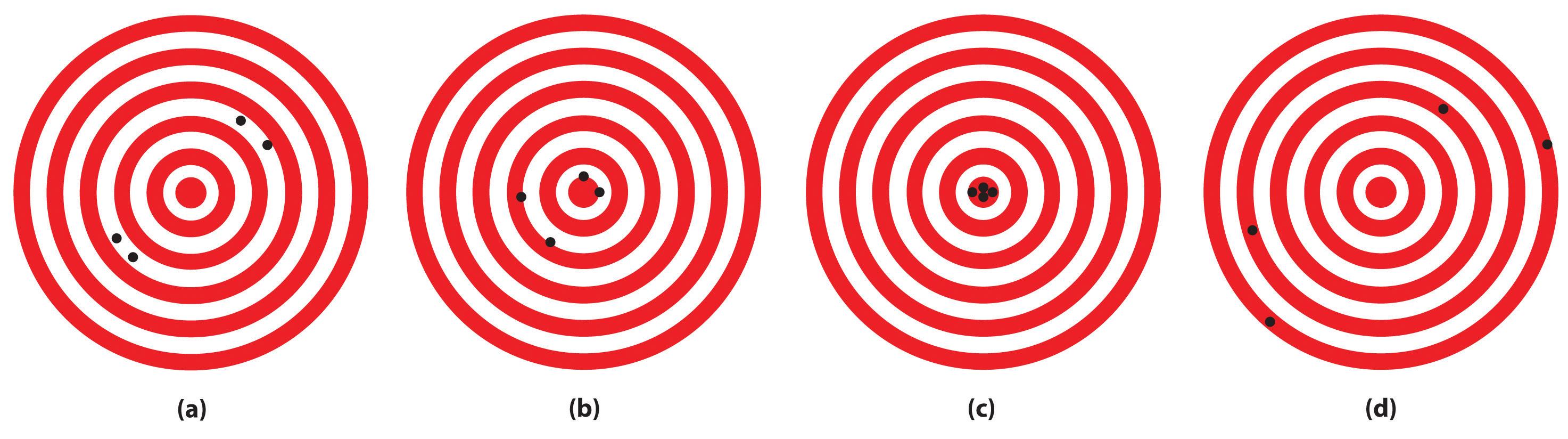Solution

1. (c)
2. (a)
3. (b)
4. (d)

### Skill Builder ES8

1. A 1-carat diamond has a mass of 200.0 mg. When a jeweler repeatedly weighed a 2-carat diamond, he obtained measurements of 450.0 mg, 459.0 mg, and 463.0 mg. Were the jeweler’s measurements accurate? Were they precise?
2. A single copper penny was tested three times to determine its composition. The first analysis gave a composition of 93.2% zinc and 2.8% copper, the second gave 92.9% zinc and 3.1% copper, and the third gave 93.5% zinc and 2.5% copper. The actual composition of the penny was 97.6% zinc and 2.4% copper. Were the results accurate? Were they precise?

Solution

1. The expected mass of a 2-carat diamond is 2 × 200.0 mg = 400.0 mg. The average of the three measurements is 457.3 mg, about 13% greater than the true mass. These measurements are not particularly accurate.

The deviations of the measurements are 7.3 mg, 1.7 mg, and 5.7 mg, respectively, which give an average deviation of 4.9 mg and a precision of

These measurements are rather precise.

2. The average values of the measurements are 93.2% zinc and 2.8% copper versus the true values of 97.6% zinc and 2.4% copper. Thus these measurements are not very accurate, with errors of −4.5% and + 17% for zinc and copper, respectively. (The sum of the measured zinc and copper contents is only 96.0% rather than 100%, which tells us that either there is a significant error in one or both measurements or some other element is present.)

The deviations of the measurements are 0.0%, 0.3%, and 0.3% for both zinc and copper, which give an average deviation of 0.2% for both metals. We might therefore conclude that the measurements are equally precise, but that is not the case. Recall that precision is the average deviation divided by the average value times 100. Because the average value of the zinc measurements is much greater than the average value of the copper measurements (93.2% versus 2.8%), the copper measurements are much less precise.